[top.htm]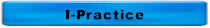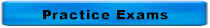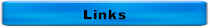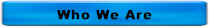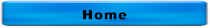Answers to More Distribution Math Questions and Charts

### You are adding 6 lbs/day of chlorine gas to a flow of 277 gpm and your total chlorine residual is 1.5 mg/L. What is your chlorine demand in mg/L?

Flow, MGD = 277 gpm ÷ 694 gpm/mgd = 0.4 MGD

Chlorine Dose, mg/L = 6 lbs/day ÷ 8.34 lbs/gal ÷ 0.4 MGD = 1.8 mg/L

Chlorine demand, mg/L = 1.8 mg/L = 1.5 mg/L = 0.3 mg/L

Click on the thumbnail below for the answers for the chart of pump station math for cylindrical tanks.

Click on the thumbnail below for the answers for the chart of pump station math for rectangular tanks.

Click on the thumbnail below for the answers for the chart of pipe math.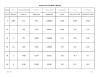Match 1 The units for pressure are A Divided by 2 Head is expressed in B 2.31 3 Velocity is measured in C 0.434 psi 4 Units of flow D psi 5 Pressure times _______ = Head E fps 6 Head __________ 2.31 = Pressure F 1440 7 20 psi = G 694 gpm 8 1 MGD = H cfs 9 A head loss of 7 feet = I 3 psi J Times K ft L 46.2 ft M Subtracted by

1 = D

2 = K

3 = E

4= H

5 = B

6 = A

7 = L

8 = G

9 = I

The formula for the volume of a rectangular tank is

A.  (Length)(Width)

B.  (0.785)(Diameter2)

C.  (Length)(Width)(Height)

D.  (0.785)(Diameter2)(Height)

The correct answer is C.  (Length)(Width)(Height)

A is the formula for the area of a rectangle

B is the formula for the area of a circle

D is the formula for the volume of a cylinder

The formula for the volume of a cylindrical tank is

A.  (Length)(Width)

B.  (0.785)(Diameter2)

C.  (Length)(Width)(Height)

D.  (0.785)(Diameter2)(Height)

The correct answer is D.  (0.785)(Diameter2)(Height)

A is the formula for the area of a rectangle

B is the formula for the area of a circle

C is the formula for the volume of a rectangle

Given a tank measures 50 feet long, 25 feet wide, and 15 ft deep, calculate:

• Surface area in ft2
• Cross-sectional area in square feet
• Volume in cf
• Capacity in gallons

• Surface area in ft2

Surface area in ft2 = Length, ft x Width, ft = 50 ft x 25 ft = 1250 ft2

• Cross-sectional area in square feet

Cross-sectional area is ft2 = Width, ft x Depth, ft = 25 ft x 15 ft = 375 square feet

• Volume in cf

Volume in cf = Length, ft x Width, ft x Depth, ft = 50 ft x 25 ft x 15 ft = 18,750 cf

• Capacity in gallons

Capacity in gallons = Vol in cf x 7.5 gal/cf = 18,750 cf x 7.5 gal/cf = 140,625 gal

For questions or comments, call 515-313-1159